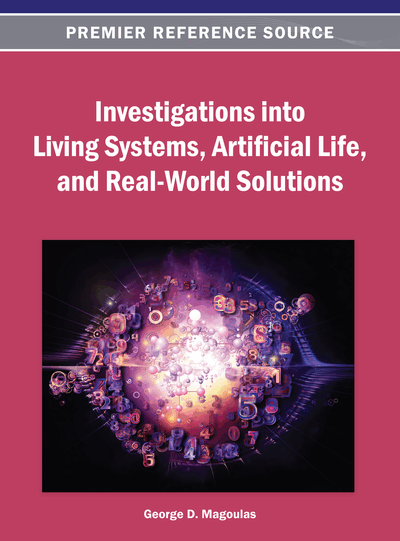# Intuitionistic Fuzzy 2-Metric Space and Some Topological Properties

Q.M. Danish Lohani (South Asian University, India)
DOI: 10.4018/978-1-4666-3890-7.ch020

## Abstract

The notion of intuitionistic fuzzy metric space was introduced by Park (2004) and the concept of intuitionistic fuzzy normed space by Saadati and Park (2006). Recently Mursaleen and Lohani introduced the concept of intuitionistic fuzzy 2-metric space (2009) and intuitionistic fuzzy 2-norm space. This paper studies precompactness and metrizability in this new setup of intuitionistic fuzzy 2-metric space.
Chapter Preview
Top

## 1. Introduction And Preliminaries

Among various developments of the fuzzy set theory (Zadeh, 1965), a progressive development has been made to find the fuzzy analogues of the classical set theory. Infact the fuzzy theory has become an area of active research for the last forty years. It has a wide range of applications in the field of science and engineering, e.g. population dynamics (Barros, Bassanezi, & Tonelli, 2000), chaos control (Fradkov & Evans, 2005), computer programming (Giles, 1980), nonlinear dynamical systems (Hong & Sun, 2006), and medicine (Barro & Marin, 2002).

Fuzzy topology is one of the most important and useful tool studied by various authors, e.g. (Erceg, 1979; Fang, 2002; Felbin, 1992; George & Veeramani, 1994; Kaleva & Seikkala, 1984; Xiao & Zhu, 2002). The most fascinating application of the fuzzy topology in quantum physics arises in e(∞)-theory due to El Naschie (1998, 2000, 2004a, 2004b, 2006a, 2006b, 2007) who presented the relation of fuzzy Kähler interpolation of e(∞) to the recent work on cosmo-topology and the Poincare dodecahedral conjecture and gave various applications and results of e(∞) theory from nano technology to brain research.

Atanassov (1986, 1994) introduced the concept of intuitionistic fuzzy sets. For intuitionistic fuzzy topological spaces, we refer to Abbas (2005), Coker (1997), Kaleva and Seikkala (1984), Lupianez (2006), and Park (2004). Recently, Saadati and Park (2006) studied the notion of intuitionistic fuzzy 2-normed spaces. Quite recently, the concept of intuitionistic fuzzy 2-normed spaces and intuitionistic fuzzy 2-normed spaces has been introduced and studied by Mursaleen and Lohani (2009) and Park (2004) respectively. Certainly there are some situations where the ordinary metric does not work and the concept of intuitionistic fuzzy metric seems to be more suitable in such cases, that is, we can deal with such situations by modelling the inexactness of the norm in some situations.

In this paper we study the concept of intuitionistic fuzzy 2-metric space which would provide a more suitable funtional tool to deal with the inexactness of the metric or 2-metric in some situations. We present here analogues of precompactness and metrizability and establish some interesting results in this new setup.

We recall some notations and basic definitions used in this paper.

## Complete Chapter List

Search this Book:
Reset